Factors Affecting Golf

Purpose: to illustrate, by a simple golf example, how the C4.5 and C4.5rules programs function.

Problem: given the training instances below, use C4.5 and C4.5rules to generate rules as to when to play, and when not to play, a game of golf.

Training Data

 Outlook Temperature Humidity Windy Play (positive) / Don't Play (negative) sunny 85 85 false Don't Play sunny 80 90 true Don't Play overcast 83 78 false Play rain 70 96 false Play rain 68 80 false Play rain 65 70 true Don't Play overcast 64 65 true Play sunny 72 95 false Don't Play sunny 69 70 false Play rain 75 80 false Play sunny 75 70 true Play overcast 72 90 true Play overcast 81 75 false Play rain 71 80 true Don't Play

The column headers - the attribute names - become part of "golf.names", the filestem.names file.
The subsequent rows - the training instances - are entered into "golf.data", the filestem.data file.

Command-Line Syntax

The following commands were executed to produce results at the default verbosity level
(% represents the prompt; it is not typed):

• % c4.5 -f golf
• % c4.5rules -f golf

"-f [filestem]" specifies the file stem to be used.

It may also be handy to redirect the output generated by these programs to files for future review using the "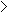" operator at the command-line as follows:

• % c4.5 -f golf > golf.dt
• % c4.5rules -f golf > golf.r

Additionally, higher verbosity levels may be specified to obtain statistical data calculated at runtime:

• e.g., C4.5 verbosity level 1
% c4.5 -f golf -v 1 > golf.dt1

• e.g., C4.5rules verbosity level 3
% c4.5rules -f golf -v 3 > golf.r3

"-v [1-3]" specifies the verbosity level to be used from a scale of 1 to 3. Omitting this switch forces C4.5 and C4.5rules to use the default verbosity level.

Finally, it is possible for both C4.5 and C4.5rules to be executed with command-line switches to encorporate test instances into the analysis, if available (see Example 3):

• e.g., C4.5 default verbosity level, using the file "golf.test"
% c4.5 -f golf -u > golf.dt

• e.g., C4.5rules verbosity level 2, using the file "golf.test"
% c4.5rules -f golf -v 2 -u > golf.r2

"-u" tells C4.5 and C4.5rules to use the unseen test instances in the "filestem.test" file following evaluation on the training data.

The following files were generated using the above commands for the purpose of illustrating the differences between the different verbosity levels:

• golf.names
• Class, attribute, and value names.
• golf.data
• Training data.
• The resulting decision tree,
• The resulting decision rules,

As you can see, higher verbosity levels provide much more quantitative data than those at the lower levels.
Since we are primarily concerned with qualitative results, however, only the generated output at the default verbosity level is examined in detail below.

Diagram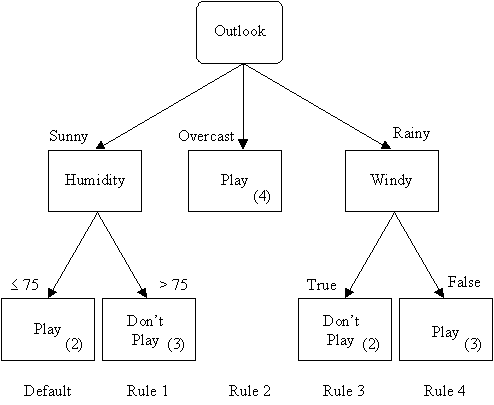Interpreting Output at the Default Verbosity Level

Preliminaries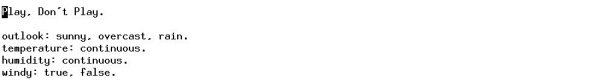golf.names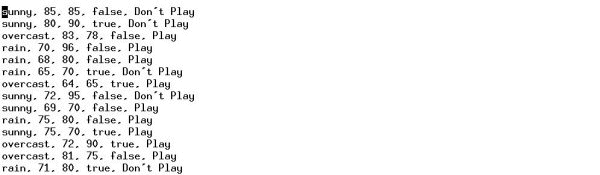golf.data

C4.5 Results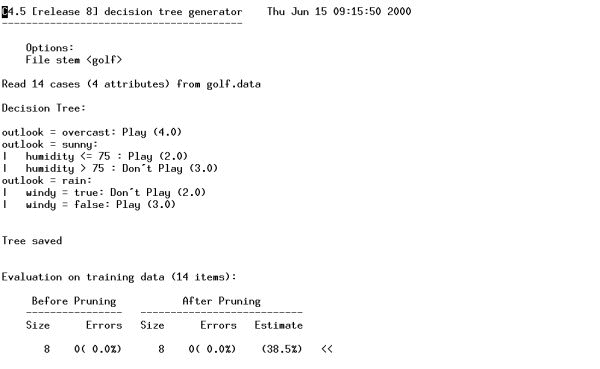The resulting decision tree.

The output generated by C4.5 at the default verbosity level is interpreted as follows:

• Firstly, the header. It indicates:
• The name of the file stem being used.
(e.g., "golf")
• The total number of training instances, or cases, read in the filestem.data file by C4.5.
(e.g., 14)
• The number of attributes per instance.
(e.g., 4)
• Secondly, one or more ASCII renditions of a generated decision tree.
• The tree consists of a spine of attribute-values that stem from a root attribute test.
• In this example, the root is the attribute test "outlook". It has three attribute-values: "sunny", "overcast", and "rain".
• Two subtrees occur: a "humidity" subtree below "sunny", and a "windy" subtree below "rain".
• The number in brackets following each leaf equals the number of training instances, out of the total number of cases presented in the header, which belong to that path in the tree.
• This number may be followed by a second number (e.g., 4.0/2.0), in which case the second value (2.0) equals the number of classification errors encountered out of the total number of classifications made from the training data in that particular path of the decision tree (4.0).
• The sum of the first series of numbers equals the total number of cases read by C4.5 from the golf.data file.
(e.g., 4.0 + 2.0 + 3.0 + 2.0 + 3.0 = 14.0)
• The sum of the second series of numbers equals the total number of errors.
(e.g., 0 for this example).
• Two binary files are created during execution:
1. filestem.unpruned: the unpruned decision tree generated and used by C4.5
2. filestem.tree: the pruned decision tree generated and used by C4.5 which is subsequently required by C4.5rules to generate rules.
• Thirdly, the unpruned decision tree and the pruned decision tree are evaluated against the training data instances to test the fitness of each.
• The first table illustrates the fitness of the unpruned tree. It has two columns:
1. Size: the size of the unpruned tree. That is, the number of nodes of which it is composed.
2. Errors: the number of classification errors and their corresponding error percentage from the total number of cases.
• The second table illustrates the fitness of the pruned tree. It has three columns:
1. Size: the size of the pruned tree. It is either less than or equal to that of the unpruned tree depending upon the extent of the pruning performed by C4.5.
2. Errors: the number of classification errors and their corresponding actual error percentage after pruning.
3. Estimate: the estimated error percentage of the tree after pruning, useful when comparing with the actual percentage.

C4.5rules Results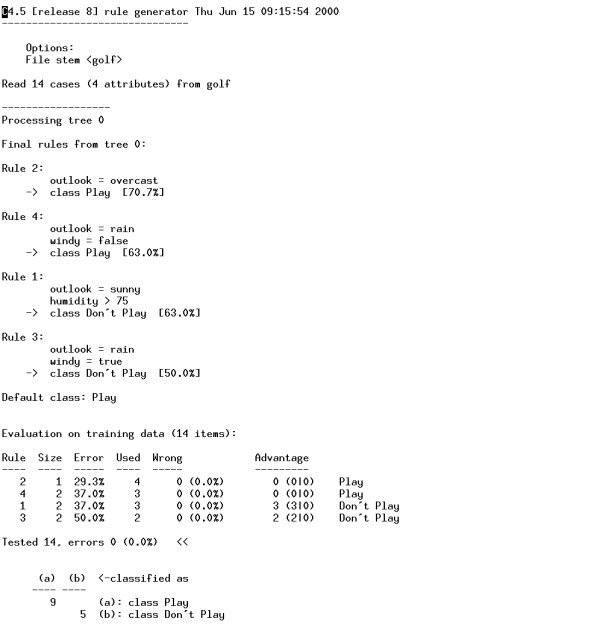The resulting decision rules.

The output generated by C4.5rules at the default verbosity level is interpreted as follows:

• Same as that of C4.5
• Secondly, the set of generated rules.
• One set of rules is generated for each pruned decision tree.
• The set of rules usually consists of at least one default rule, which is used to classify unseen instances when no other rule applies.
(e.g., Play)
• Every enumerated rule is composed of attribute-values and a resulting classification, followed by a percentage which represents the accuracy of that rule.
(e.g., Rule 1: if "outlook = sunny" but "humidity > 75" then "Don't Play";
according to C4.5rules, this rule is accurate 63% of the time, and thus has a 37% error margin)
• Thirdly, the rules are evaluated against the training data instances to test the fitness of each.
• The rule table has six columns:
1. Rule: the number assigned by C4.5rules to each rule.
2. Size: the size of the rule. That is, the number of antecedents of which it is composed.
3. Error: the error margin of the rule.
4. Used: the number of times the rule was used, regardless of correctness, in classifying the training instances. The sum of this column yields the total number of cases.
5. Wrong: the number of times a rule has been misused, and the corresponding percentage of this value from the previous column.
6. Advantage: the difference between the number of times a rule has been used correctly and the number of times it has been used incorrectly.
• For example, suppose a rule had the following values for the table:

 Used Wrong Advantage 4 1(25.0% = 1 wrong / 4 used) 2 (3 | 1)

• Wrong = 1 = the number of times the rule has been used incorrectly.
• Right = 3 = the number of times the rule has been used correctly (4 total used - 1 wrong).
• Advantage = 2 = the difference between 3 rights and 1 wrong.

Thus, advantage is an overall measure of the effectiveness of a rule.

• The table also sums up the total number of wrong classifications and calculates the percentage error from the total number of cases tested.
• Fourthly, C4.5rules sums up the number of correct and incorrect classifications in a table. Both the rows and columns have the same headers, but there is a distinction between them:
• The rows of the table are the classes available for use in the classification process.
• The columns of the table are the classes chosen during classification.
• The cell where a particular row and column intersect may either contain a number or not.
• If the cell does not contain a number, then no tested instances under that cell's row class have been classified by its corresponding column class.
• Otherwise, the number represents the number of instances of the row class which have been classified as a member of the corresponding column class.
• Misclassifications occur when the row and column classes of a cell do not match.
• For example, in the table below:

 (a) (b)classified as 9 (a): class Play 5 (b): class Don't Play

9 instances of the known class "Play" were correctly classified using the generated rules as members of class "Play".
5 instances of the known class "Don't Play" were correctly classified using the generated rules as members of class "Don't Play".
0 instances were incorrectly classified.
9 + 5 = 14 = the total number of instances tested.

Summary

Rule 1 suggests that if "outlook = sunny" and "humidity > 75" then "Don't Play".
Rule 2 suggests that if "outlook = overcast" then "Play".
Rule 3 suggests that if "outlook = rain" and "windy = true" then "Don't Play".
Rule 4 suggests that if "outlook = rain" and "windy = false" then "Play".
Otherwise, "Play" is the default class.